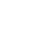Live chatToyota and ford are two strong competitors in the automobile industry. Toyota is a major automobile manufacturer in the world with a good performance all over the globe. Its leading market is Africa where it dominates in number vehicles imported and a good local market in Japan. Their financial ratios are as follows:

## Toyota financial ratios

P/E ratio (Price-Earnings Ratio)

P/E ratio=14.83

A valuation ratio of a company's current share price compared to its per-share earnings. EPS is normally calculated from the previous four quarters, but can also be calculated from the estimates expected in the following four quarters. A third variation is derived from the last two real quarters and the estimates of the following two quarters.

Calculated as:

A figure of 14.83 means that the company has high returns on capital, implying that the company's net income is growing

## Return on assets

Return on assets= 1.8%.This an indicator of profitable gain for the firm. It is valuation ratio of a company's current share price in comparison to its per-share earnings.

The formula for return on assets is:

Return on invested capital

Return on invested capital =3.0%.

Such a percentage is a record of increased income from the firm's transactionsThe formula for ROIC is as follows:

## SG&A as percentage of revenue

SG&A as percentage of revenue =10.6%

SG&A includes expenses related to marketing, promoting, and distribution of goods and services. This ratio is important in analyzing how well revenue and costs are managed in a firm. Toyota's figure shows that its operating income is viable.

Ford

P/E ratio (Price-Earnings Ratio)

P/E ratio=12.83.

This shows that the firm's P/E ratio was lower than that of Toyota.

## Return on assets

Returns on assets =4.1%.

It indicates a gain in the firm's income.

## Return on invested capital

Return on invested capital =6.4%.

Return on invested capital gives the income of the on an investment. It shows that

R\$D as % of revenue

R\$D as a % of revenue =1.0%.

It shows the returns of the firm as percentage of the revenue. This figure implies that ford is currently profitable.

0

Preparing Orders

0

Active Writers

0%

Positive Feedback

0

Support Agents

Limited offerget 15% off your 1st order with code first15